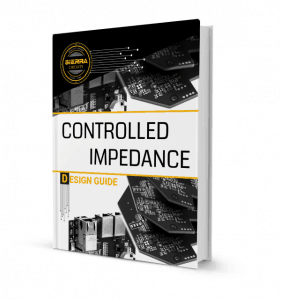## PCB Transmission Line: Critical Length, Controlled Impedance and Rise/Fall Time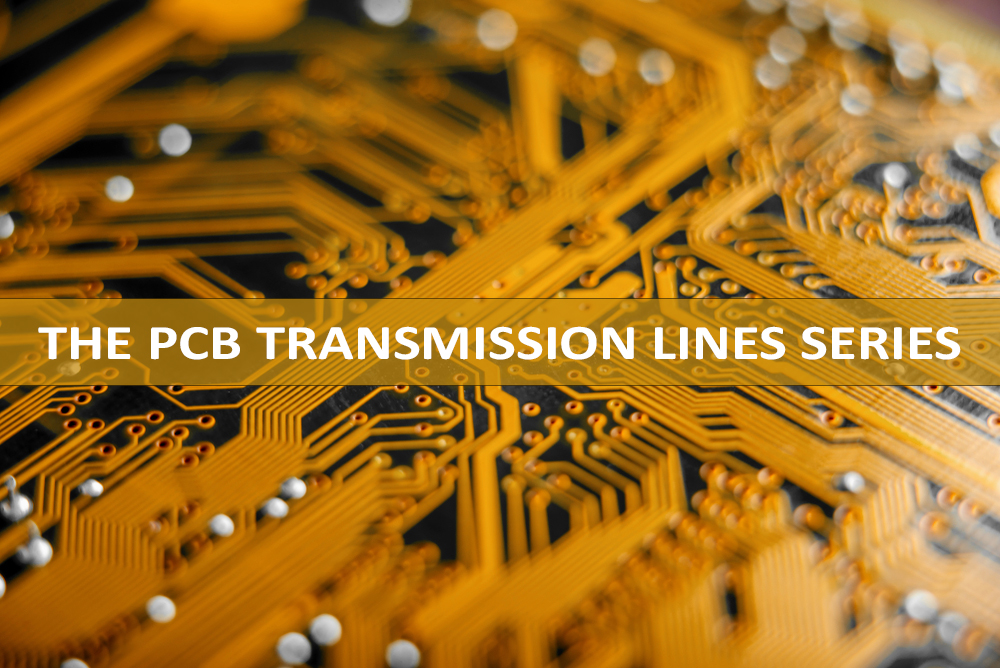After explaining the importance of the signal speed and propagation delay in a PCB transmission line, we are now going to talk about the transmission line effects, the critical length, the rise/fall time and when the length of an interconnection is to be considered as a controlled impedance transmission line.

As we mentioned in our article about what a PCB transmission line is, for high-speed or high-frequency signals, we need to consider the transmission line effects. We can use a few thumb rules:

In case of high-frequency analog signals, let the maximum frequency content in the signal =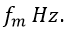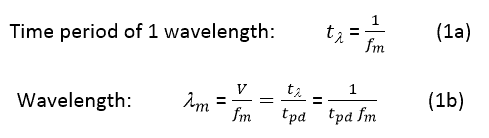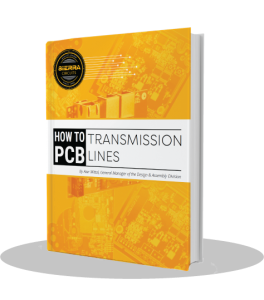#### Critical length lc

For analog signals, the critical length lc is defined as one-fourth of the wavelength of the highest signal frequency contained in the signal.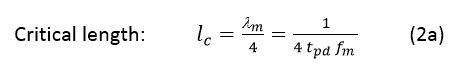In case of digital signals, the fastest rise/fall time of a signal pulse is the most important parameter. It defines the transition time from one logic level to another logic level. Basically, the transition time of the data bit. For digital signals, the critical length lc is defined as the line length over which the signal propagation time is half of the fastest rise/fall time of the signal pulses.

If tr = fastest rise/fall time of the digital signal, the time of the propagation of the signal over the length lc is tpd (propagation delay). lc = tr/2 (by definition of lc).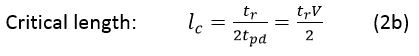This definition implies that the signal should be able to travel from the source over a length lc of the line. And then return over the same length lc of the line back to the source point in a total time equal to the rise/fall time tr.

Equations 2a and 2b above are related if we consider the highest frequency content in a digital signal rise/fall time.

The highest frequency content in a digital signal of rise/fall time tr is given by (as per Fourier Analysis):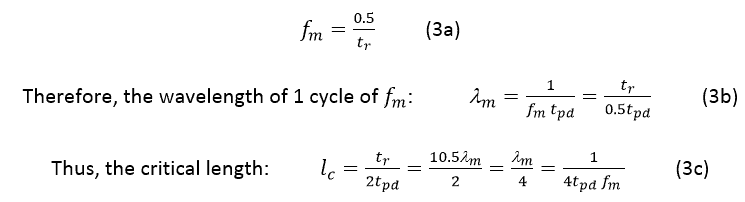Which is the same as Equation 2a above.

#### Shortline

If the line length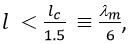there is a shortline and it is not necessary to consider its transmission line effects, nor to design it as a controlled impedance line.

But if the line length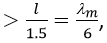it then becomes necessary to consider the transmission line effects and to design such lines as controlled impedance lines.

Example:

If the fastest signal rise/fall time is: tr = 1ns, then, assuming the FR4 material with a dielectric constant Er = 4,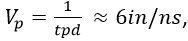and the critical length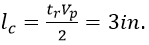Therefore, signal traces longer than 3/1.5 = 2 inches need to be designed as controlled impedance lines. Note here that tr of 1ns corresponds to a maximal signal frequency: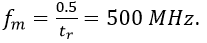Estimate the rise/fall time from data transfer rate (DTR) or clock frequency

The data transfer rate (DTR) is measured in bits per second (bps or bits/sec or b/s). And the clock frequency (Fclock) in Hz.

DTR is usually ≥ 2 Fclock. Henceforth, it will be safe to use the following rule: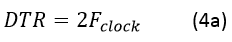If we don’t know the signal rise/fall time, we can use the following rule: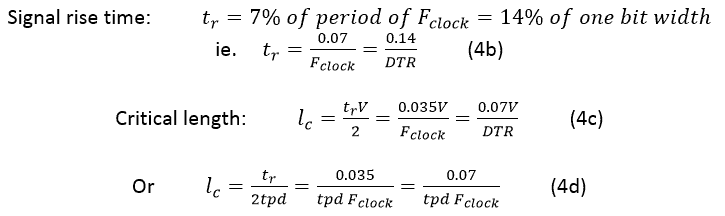Example:

For Fclock = 1GHz or DTR = Gbps, and for a PCB material with Er = 4, we get: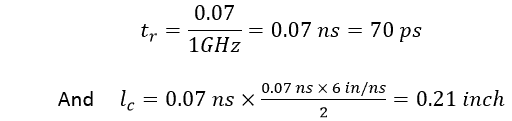#### 3 dB bandwidth

For a signal with rise/fall time tr, the 3 dB bandwidth is given by the following rule: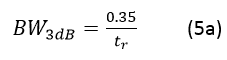Therefore, for a clock of frequency Fclock, we get: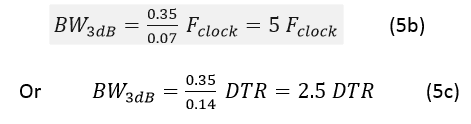Next week, we will explain how to analyze a PCB transmission line using quantities, such as voltages and currents, and line parameters, such as resistance, inductance, capacitance, and conductance.